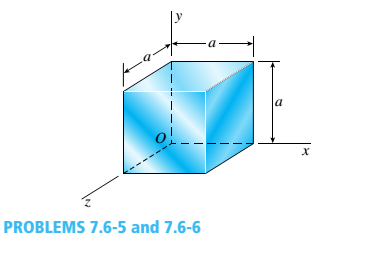# Solve the preceding problem if the cube is granite (E = 80 GPa, v = 0.25) with dimensions E = 89 mm and compressive strains E = 690 X l0-6 and = = 255 X 10-6. For part (c) of Problem 7.6-5. find the maximum value of cr when the change in volume must be limited to 0.11%. For part. find the required value of when the strain energy must be 33 J.### Mechanics of Materials (MindTap Co...

9th Edition
Barry J. Goodno + 1 other
Publisher: Cengage Learning
ISBN: 9781337093347
Chapter 7, Problem 7.6.6P
Textbook Problem
1 views

## Solve the preceding problem if the cube is granite (E = 80 GPa, v = 0.25) with dimensions E = 89 mm and compressive strains E = 690 X l0-6 and = = 255 X 10-6. For part (c) of Problem 7.6-5. find the maximum value of cr when the change in volume must be limited to 0.11%. For part. find the required value of when the strain energy must be 33 J.(a)

To determine

The normal stresses acting on the x , y and z faces of the cube.

### Explanation of Solution

Given information:

A cube of cast iron having side 89 mm is tested under triaxial stress. The strain in the x direction is 690 × 10 6 , strain in y direction and z direction is being equal which is 255 × 10 6 . The modulus of elasticity is 80 GPa and the Poisson’s ratio is 0.25 .

Explanation:

Write the expression for the stress along x axis.

σ x = E ( 1 + ν ) ( 1 2 ν ) [ ( 1 ν ) ε x + ν ( ε y + ε z ) ] ...... (I)

Here, the stress alon x axis is σ x , modulus of elasticity is E , the Poisson’s ratio is ν , strain along x axis is ε x , strain along y axis is ε y and strain along z axis is ε z .

Write the expression for stress along y axis.

σ y = E ( 1 + ν ) ( 1 2 ν ) [ ( 1 ν ) ε y + ν ( ε x + ε z ) ] ...... (II)

Here, stress along y axis is σ y .

Write the expression for stress along z axis.

σ z = E ( 1 + ν ) ( 1 2 ν ) [ ( 1 ν ) ε z + ν ( ε x + ε y ) ] ...... (III)

Here, stress along z axis is σ z .

Calculation:

Substitute 690 × 10 6 for ε x , 255 × 10 6 for ε y , 255 × 10 6 for ε z , 80 GPa for E and 0.25 for ν in Equation (I).

σ x = 80 GPa ( 1 + 0.25 ) ( 1 ( 2 × 0.25 ) ) [ ( 0.75 × 690 ) + ( 0.25 × ( 255 255 ) ) ] × 10 6 = 128 GPa ( 645 × 10 6 ) = 82560 GPa × ( 10 3 MPa 1 GPa ) × 10 6 = 82

(b)

To determine

The maximum shear stress in the material.

(c)

To determine

The change in the volume of the cube.

(d)

To determine

The strain energy stored in the cube.

(e)

To determine

The maximum value of normal stress along the x axis.

(f)

To determine

The required value of strain along the x axis.

### Still sussing out bartleby?

Check out a sample textbook solution.

See a sample solution

#### The Solution to Your Study Problems

Bartleby provides explanations to thousands of textbook problems written by our experts, many with advanced degrees!

Get Started

Find more solutions based on key concepts
What factors might determine when a hole must be finished by reaming?

Precision Machining Technology (MindTap Course List)

Identify and describe the basic components of a DSS.

Fundamentals of Information Systems

What is the typical key size of a strong encryption system used on the Web today?

Principles of Information Security (MindTap Course List)

A manufacturer of loose-fill cellulose insulating material provides a table showing the relationship between th...

Engineering Fundamentals: An Introduction to Engineering (MindTap Course List)

What is a common problem in calculating start and finish times? Provide an example.

Systems Analysis and Design (Shelly Cashman Series) (MindTap Course List)

A(n) _____ database stores data in tables that consist of rows and columns. (508) a. object-oriented b. multidi...

Enhanced Discovering Computers 2017 (Shelly Cashman Series) (MindTap Course List)

Name five different types of welding career opportunities.

Welding: Principles and Applications (MindTap Course List)

If your motherboard supports ECC DDR3 memory, can you substitute non-ECC DDR3 memory?

A+ Guide to Hardware (Standalone Book) (MindTap Course List)